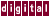In general, when KAP inserts an iteration-local temporary, the last value assigned to that temporary must be reassigned to the original scalar. When `/optimize` is set to 2 or higher, KAP performs lifetime analysis to determine if the value of the original scalar is used outside the loop.

If the variable is reused within the compilation unit, the last value of the scalar is assigned, as shown in the following example:

```for ( i=0; i<n; i++ ) {
x = a[i];
y = b[i];
c[i] = x + y;
}
printf(" x=%f \n",x);
```

Becomes:

```_Kii1 = n;
for ( i = 0; i<n; i++ ) {
c[i] = a[i] + b[i];
}
if (_Kii1 > 0)
x = a[_Kii1-1];
printf( " x=%f \n",x);
```

Previous Page Next Page Contents Index
Command-Line Qualifiers